### A general principle for the construction of points of the intersection of cones and cylinders

 To construct the points of the intersection of a cone and a cylinder we choose cutting planes that intersect both surfaces along their generatrices. A plane through the vertex of a cone intersects that cone in a generatrix and a plane parallel with the axis of a cylinder intersects that cylinder in its generatrix. Therefore, the system of planes that intersect both surfaces in their generatrices is a pencil of planes that contain the line through the vertex of the cone parallel to the axis of the cylinder. Every plane of that pencil intersects both the cone and the cylinder in two generatrices. Four points which are intersections of these four lines, different from the vertices of the surfaces, are points on the curve of intersection. right-click $$\rightarrow$$ PLAY

### Examples of the intersection of a cone and a cylinder

#### Proper curves of the 4th order

 If one surface passes only partially through the other surface, then the intersection curve is a one-branch curve and we say this is a partial intersection. If every generatrix of the cylinder intersect the cone in two real and different points or every generatrix of the cone intersects the cylinder in two real and different points, then the intersection curve is two-branch and such intersection is called a complete intersection.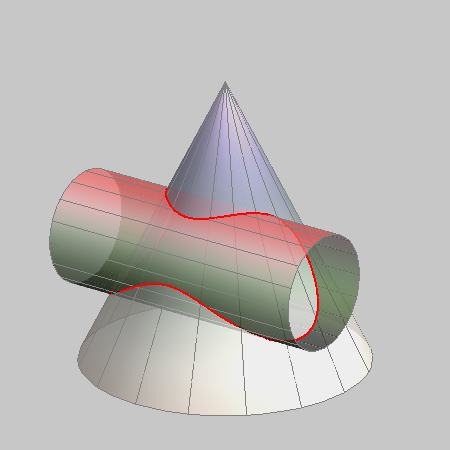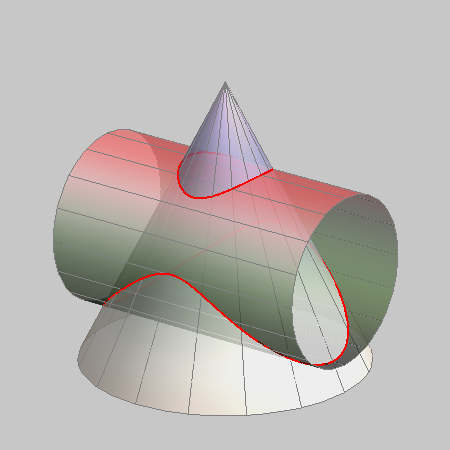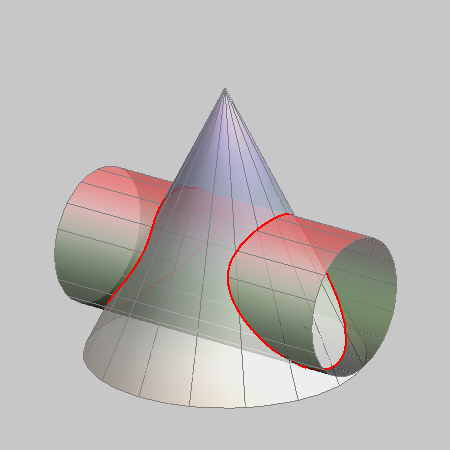PARTIAL INTERSECTION - a one-branch curve COMPLETE INTERSECTION - a two-branch curve

 As we have seen while studying intersections of two cones, a double point of the intersection curve is obtained in two cases. First is when the cone and the cylinder have the same tangent plane at a regular point of both surfaces and the second is when the cylinder passes through the double point of the cone, its vertex.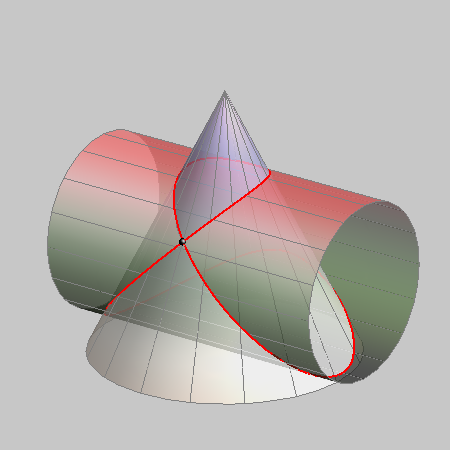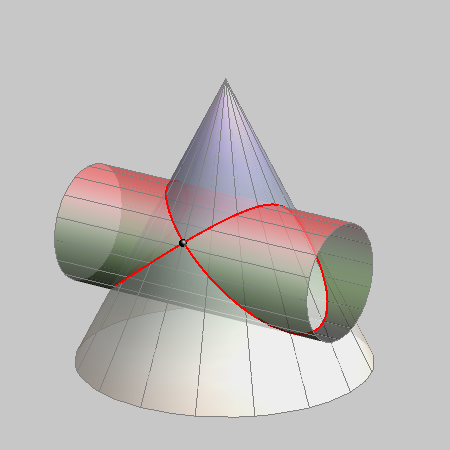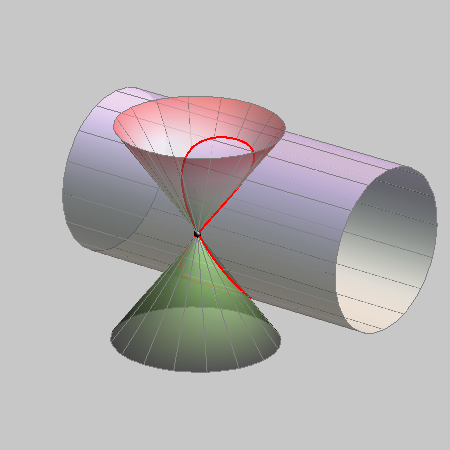intersection curve with one DOUBLE POINT The cone and the cylinder have the same tangent plane. intersection curve with one DOUBLE POINT The cylinder passes through the vertex of the cone.

#### Degenerated curves of the 4th order

Intersection curve of a cone and a cylinder can never degenerate into 4 lines because their vertices cannot coincide (vertex of a cone cannot be a point at infinity).
Possible degenerations of the intersection curve of a cone and a cylinder are: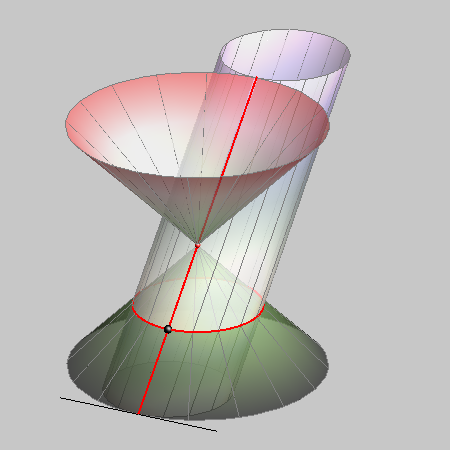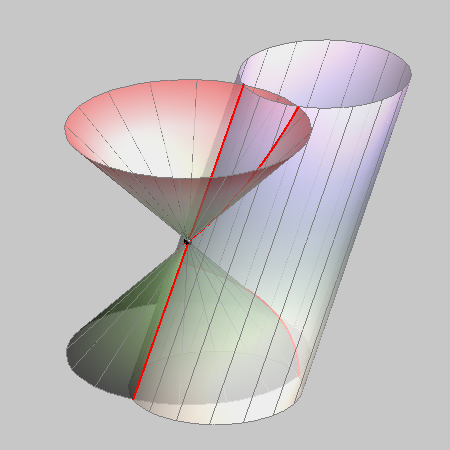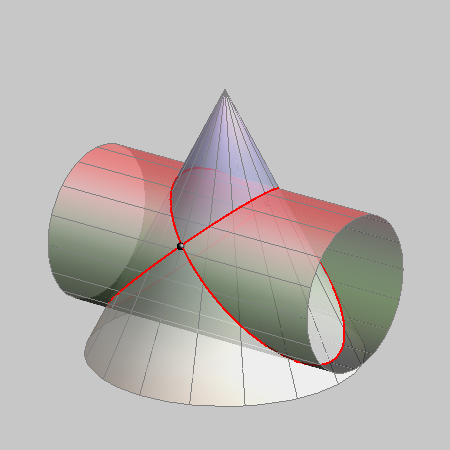a double line and a conic The cone and the cylinder have a common generatrix and the same tangent plane along that generatrix. a line and a curve of the 3rd order The cone and the cylinder have a common generatrix but the tangent planes along that generatrix are different. two conics The cone and the cylinder do not have a common generatrix but the tangent planes are the same at two points on the intersection of surfaces.

Created by Sonja Gorjanc, translated by Helena Halas and Iva Kodrnja - 3DGeomTeh - Developing project of the University of Zagreb